Balbharati Maharashtra State Board 11th Commerce Maths Solution Book Pdf Chapter 9 Differentiation Miscellaneous Exercise 9 Questions and Answers.

## Maharashtra State Board 11th Commerce Maths Solutions Chapter 9 Differentiation Miscellaneous Exercise 9

I. Differentiate the following functions w.r.t.x.

Question 1.
x5
Solution:
Let y = x5
Differentiating w.r.t. x, we get
$$\frac{\mathrm{d} y}{\mathrm{~d} x}=\frac{\mathrm{d}}{\mathrm{d} x} x^{5}=5 x^{4}$$

Question 2.
x-2
Solution:
Let y = x-2
Differentiating w.r.t. x, we get
$$\frac{\mathrm{d} y}{\mathrm{~d} x}=\frac{\mathrm{d}}{\mathrm{d} x}\left(x^{-2}\right)=-2 x^{-3}=\frac{-2}{x^{3}}$$

Question 3.
√x
Solution:
Let y = √x
Differentiating w.r.t. x, we get
$$\frac{\mathrm{d} y}{\mathrm{~d} x}=\frac{\mathrm{d}}{\mathrm{d} x} \sqrt{x}=\frac{1}{2 \sqrt{x}}$$Question 4.
x√x
Solution:
Let y = x√x
∴ y = $$x^{\frac{3}{2}}$$
Differentiating w.r.t. x, we get
$$\frac{\mathrm{d} y}{\mathrm{~d} x}=\frac{\mathrm{d}}{\mathrm{d} x} x^{\frac{3}{2}}=\frac{3}{2} x^{\frac{1}{2}}$$

Question 5.
$$\frac{1}{\sqrt{x}}$$
Solution:
Let y = $$\frac{1}{\sqrt{x}}$$
∴ y = $$x^{\frac{-1}{2}}$$
Differentiating w.r.t. x, we get
$$\frac{\mathrm{d} y}{\mathrm{~d} x}=\frac{-1}{2} x^{\frac{-3}{2}}=\frac{-1}{2 x^{\frac{3}{2}}}$$

Question 6.
7x
Solution:
Let y = 7x
Differentiating w.r.t. x, we get
$$\frac{\mathrm{d} y}{\mathrm{~d} x}=\frac{\mathrm{d}}{\mathrm{d} x} 7^{x}=7^{x} \log 7$$

II. Find $$\frac{d y}{d x}$$ if

Question 1.
y = x2 + $$\frac{1}{x^{2}}$$
Solution: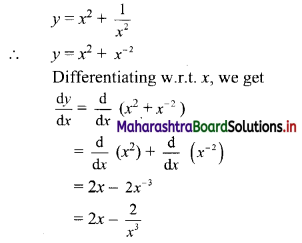Question 2.
y = (√x + 1)2
Solution: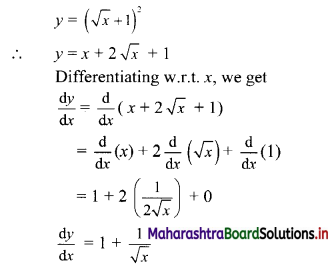Question 3.
y = $$\left(\sqrt{x}+\frac{1}{\sqrt{x}}\right)^{2}$$
Solution: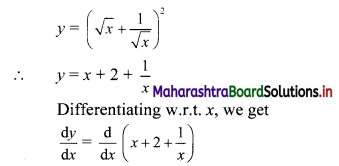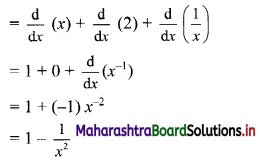Question 4.
y = x3 – 2x2 + √x + 1
Solution: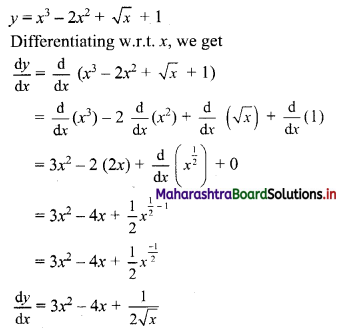Question 5.
y = x2 + 2x – 1
Solution: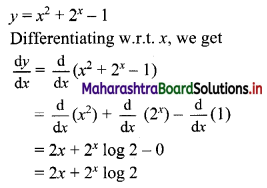Question 6.
y = (1 – x)(2 – x)
Solution: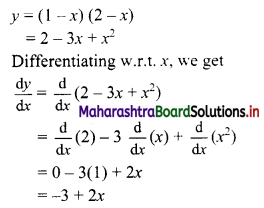Question 7.
y = $$\frac{1+x}{2+x}$$
Solution: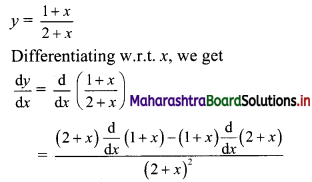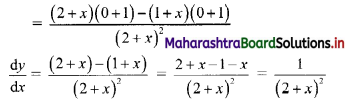Question 8.
y = $$\frac{(\log x+1)}{x}$$
Solution: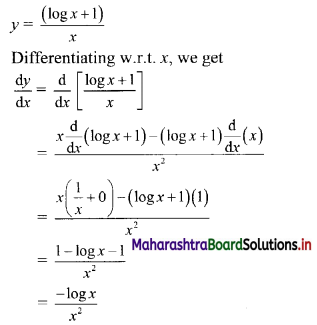Question 9.
y = $$\frac{e^{x}}{\log x}$$
Solution: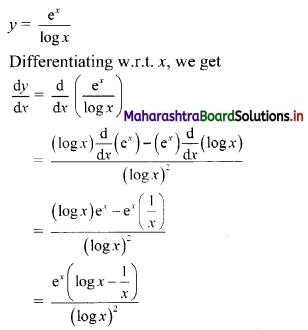Question 10.
y = x log x (x2 + 1)
Solution: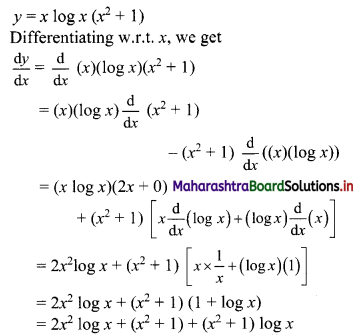III. Solve the following:

Question 1.
The relation between price (P) and demand (D) of a cup of Tea is given by D = $$\frac{12}{P}$$. Find
the rate at which the demand changes when the price is ₹ 2/-. Interpret the result.
Solution:
Demand, D = $$\frac{12}{P}$$
Rate of change of demand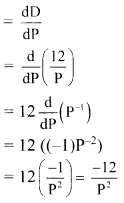When price P = 2,
Rate of change of demand,
$$\left(\frac{\mathrm{dD}}{\mathrm{dP}}\right)_{\mathrm{P}=2}=\frac{-12}{(2)^{2}}=-3$$
∴ When the price is 2, the rate of change of demand is -3.
∴ Here, the rate of change of demand is negative demand would fall when the price becomes ₹ 2.

Question 2.
The demand (D) of biscuits at price P is given by D = $$\frac{64}{P^{3}}$$, find the marginal demand
when the price is ₹ 4/-.
Solution:
Given demand D = $$\frac{64}{P^{3}}$$
Now, marginal demand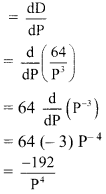When P = 4
Marginal demand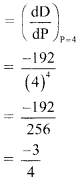Question 3.
The supply S of electric bulbs at price P is given by S = 2p3 + 5. Find the marginal supply when the price is ₹ 5/-. Interpret the result.
Solution:
Given, supply S = 2p3 + 5
Now, marginal supply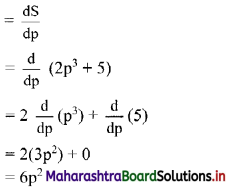∴ When p = 5
Marginal supply = $$\left(\frac{\mathrm{dS}}{\mathrm{dp}}\right)_{\mathrm{p}=5}$$
= 6(5)2
= 150
Here, the rate of change of supply with respect to the price is positive which indicates that the supply increases.

Question 4.
The total cost of producing x items is given by C = x2 + 4x + 4. Find the average cost and the marginal cost. What is the marginal cost when x = 7?
Solution:
Total cost C = x2 + 4x + 4
Now. Average cost = $$\frac{C}{x}=\frac{x^{2}+4 x+4}{x}$$
= x + 4 + $$\frac{4}{x}$$
and Marginal cost = $$\frac{\mathrm{dC}}{\mathrm{d} x}=\frac{\mathrm{d}}{\mathrm{d} x}$$(x2 + 4x + 4)
= $$\frac{\mathrm{d}}{\mathrm{d} x}$$ (x2) + 4$$\frac{\mathrm{d}}{\mathrm{d} x}$$ (x) + $$\frac{\mathrm{d}}{\mathrm{d} x}$$ (4)
= 2x + 4(1) + 0
= 2x + 4
∴ When x = 7,
Marginal cost = $$\left(\frac{\mathrm{d} \mathrm{C}}{\mathrm{d} x}\right)_{x=7}$$
= 2(7) + 4
= 14 + 4
= 18

Question 5.
The demand D for a price P is given as D = $$\frac{27}{P}$$, find the rate of change of demand when the price is ₹ 3/-.
Solution:
Demand, D = $$\frac{27}{P}$$
Rate of change of demand = $$\frac{dD}{dP}$$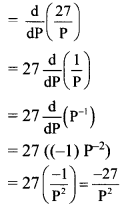When price P = 3,
Rate of change of demand,
$$\left(\frac{\mathrm{dD}}{\mathrm{dP}}\right)_{\mathrm{P}=3}=\frac{-27}{(3)^{2}}=-3$$
∴ When price is 3, Rate of change of demand is -3.Question 6.
If for a commodity; the price demand relation is given as D = $$\left(\frac{P+5}{P-1}\right)$$. Find the marginal demand when price is ₹ 2/-
Solution: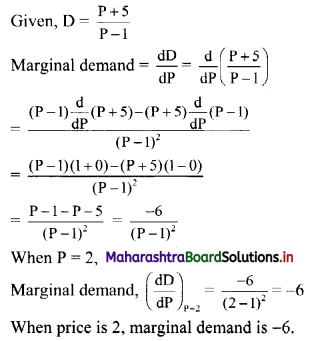Question 7.
The price function P of a commodity is given as P = 20 + D – D2 where D is demand. Find the rate at which price (P) is changing when demand D = 3.
Solution:
Given, P = 20 + D – D2
Rate of change of price = $$\frac{dP}{dD}$$
= $$\frac{d}{dD}$$(20 + D – D2)
= 0 + 1 – 2D
= 1 – 2D
Rate of change of price at D = 3 is
$$\left(\frac{\mathrm{dP}}{\mathrm{dD}}\right)_{\mathrm{D}=3}$$ = 1 – 2(3) = -5
∴ Price is changing at a rate of -5, when demand is 3.

Question 8.
If the total cost function is given by C = 5x3 + 2x2 + 1; find the average cost and the marginal cost when x = 4.
Solution:
Total cost function C = 5x3 + 2x2 + 1
Average cost = $$\frac{C}{x}$$
= $$\frac{5 x^{3}+2 x^{2}+1}{x}$$
= 5x2 + 2x + $$\frac{1}{x}$$
When x = 4,
Average cost = 5(4)2 + 2(4) + $$\frac{1}{4}$$
= 80 + 8 + $$\frac{1}{4}$$
= $$\frac{320+32+1}{4}$$
= $$\frac{353}{4}$$
Marginal cost = $$\frac{\mathrm{dC}}{\mathrm{d} x}$$
= $$\frac{d}{dx}$$ (5x3 + 2x2 + 1)
= 5$$\frac{d}{dx}$$ (x3) + 2 $$\frac{d}{dx}$$ (x2) + $$\frac{d}{dx}$$ (1)
= 5(3x2) + 2(2x) + 0
= 15x2 + 4x
When x = 4, marginal cost = $$\left(\frac{\mathrm{dC}}{\mathrm{d} x}\right)_{x=4}$$
= 15(4)2 + 4(4)
= 240 + 16
= 256
∴ The average cost and marginal cost at x = 4 are $$\frac{353}{4}$$ and 256 respectively.Question 9.
The supply S for a commodity at price P is given by S = P2 + 9P – 2. Find the marginal supply when the price is 7/-.
Solution:
Given, S = P2 + 9P – 2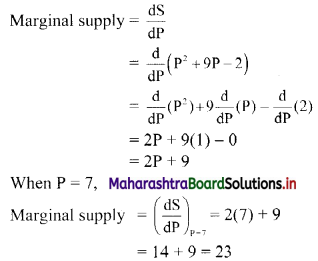∴ The marginal supply is 23, at P = 7.

Question 10.
The cost of producing x articles is given by C = x2 + 15x + 81. Find the average cost and marginal cost functions. Find the marginal cost when x = 10. Find x for which the marginal cost equals the average cost.
Solution:
Given, cost C = x2 + 15x + 81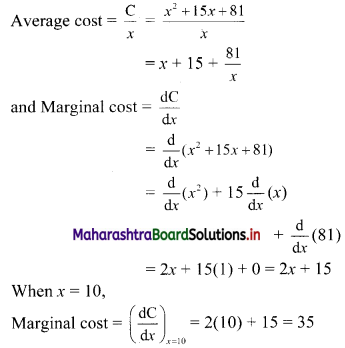If marginal cost = average cost, then
2x + 15 = x + 15 + $$\frac{81}{x}$$
∴ x = $$\frac{81}{x}$$
∴ x2 = 81
∴ x = 9 …..[∵ x > 0]